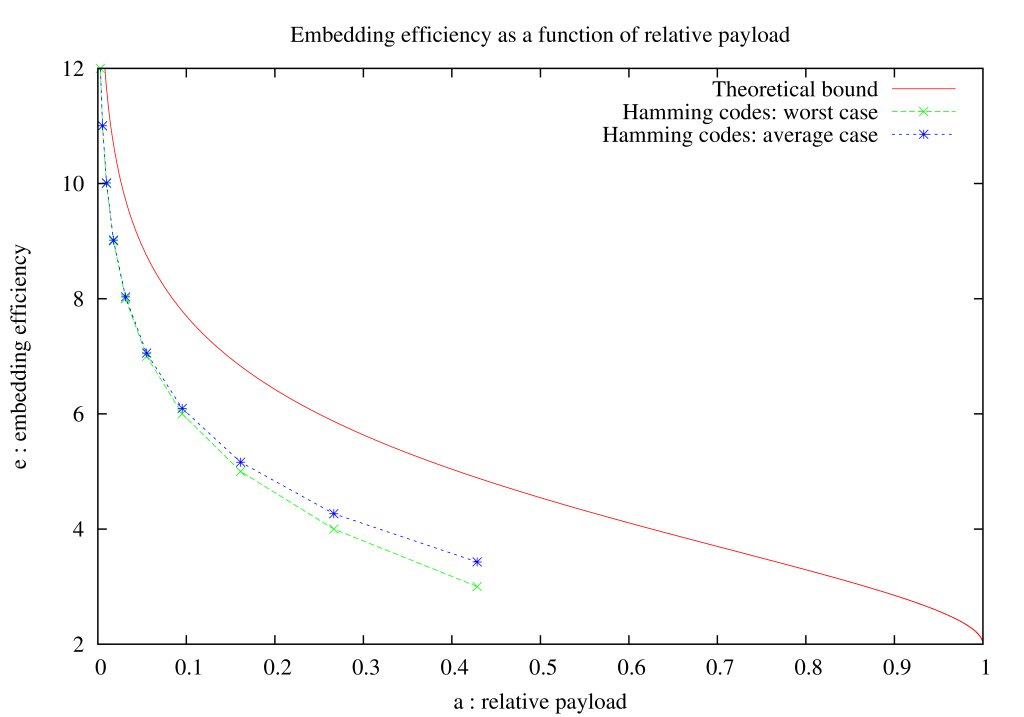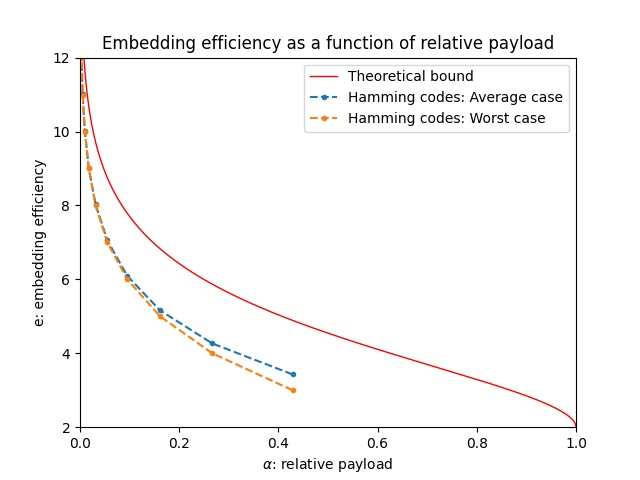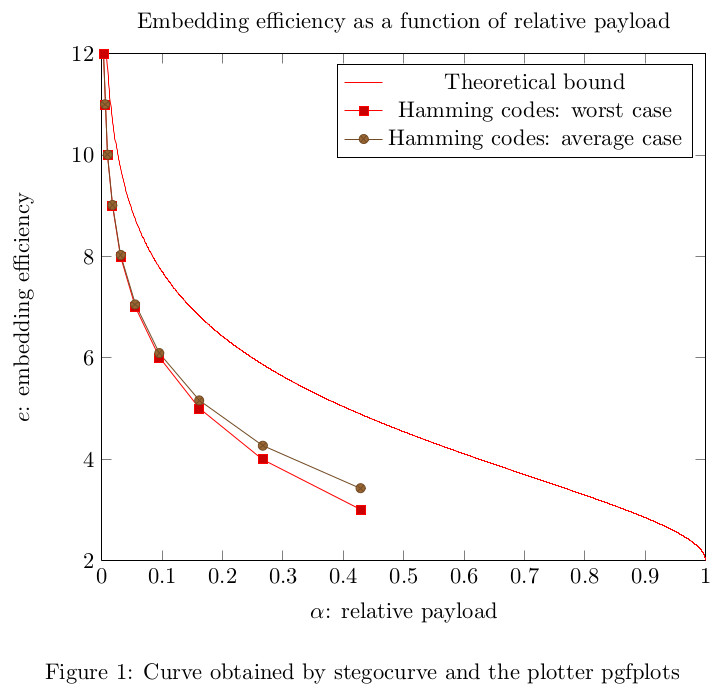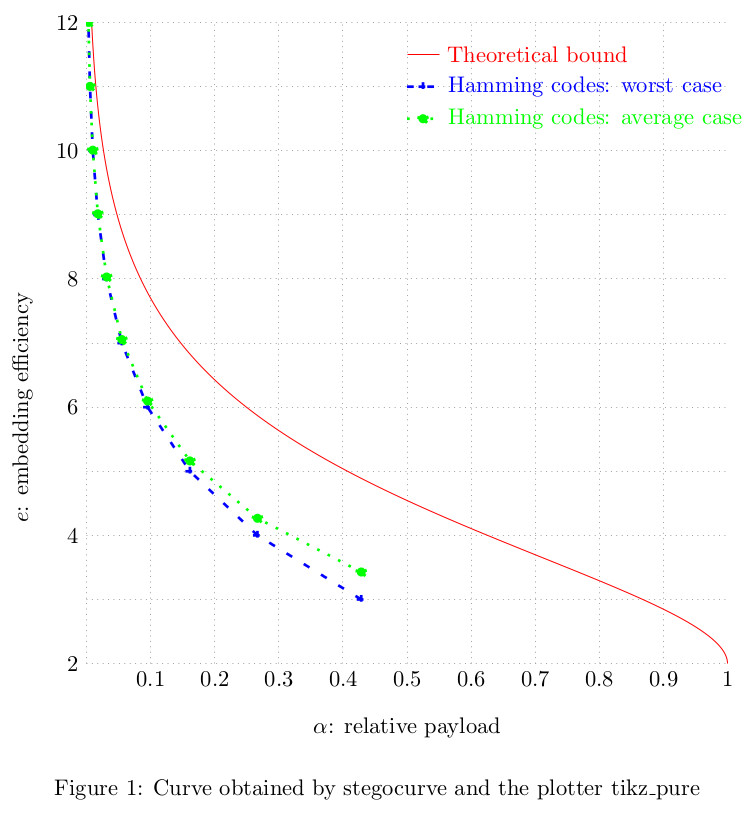stegocurve  1.2 Tool for plot easily the efficiency of code based steganography
stegocurve Documentation
Date
2023-03-21
Version
1.2

stegocurve is free software: you can redistribute it and/or modify it under the terms of the GNU General Public License as published by the Free Software Foundation, either version 3 of the License, or (at your option) any later version.

stegocurve is distributed in the hope that it will be useful, but WITHOUT ANY WARRANTY; without even the implied warranty of MERCHANTABILITY or FITNESS FOR A PARTICULAR PURPOSE. See the GNU General Public License for more details.

You should have received a copy of the GNU General Public License along with stegocurve. If not, see http://www.gnu.org/licenses/.

# Description

This tool is provided to help researcher to plot easily the embedding efficiency as a function of relative payload for code based steganography; as a fronted of gnuplot, pgfplots or tikz.

# How to use it?

## Compilation

The tool stegocurve was developped in ANSI-C from scratch. The compilation is done with a makefile. We can use the command make in following way :

\$ make

Then stegocurve will be compiled and the running file will be in the directory ./bin/ . Then to running it, run :

\$ ./bin/stegocurve

The makefile propose the following option:

• doc : generate all the documentation thanks to Doxygen. The documentation files are available in the directory doc in html form.
• all : compile the project and the full documentation.
• clean : remove all object and temporary files (.o, *~).
• distclean : is equivalent to clean and remove the running file ./bin/stegocurve
• tarball : is equivalent to distclean and make a archive in .tar.gz in the parent directory.

## Execution

Plot curve of efficiency of code based steganography need two step:

• generate the data file;
• create a gnuplot file and plot in eps and pdf formats.

It is why there is two possible actions with stegocurve. The first one is the generation of data files.

where DataDirectory is a mandatory option and indicates where the data files will be created. If the directory DataDirectory does not exist, then it will be created. Otherwise, if it exists with old data files, then they will be removed by the new ones.
The second possible action with stegocurve is plotting the needed curves with:

\$ ./bin/stegocurve PLOTTER datafile1 name1 ... datafileN nameN outputname

where the following options are mandatory:

• PLOTTER is the plotter choice, the possible values are gnuplot, mathplotlib, pgfplots and tikz;
• datafilei the data file generated by the action generate of stegocurve ;
• namei the name of the curve in the plot of the previous data file;
• outputname the name of the output file where will be plot the data files.

### Error

When an error occur in the argument sequence, the following message will appear, in stderr, which is usually the current terminal:

\$ ./bin/stegocurve
USAGE: stegocurve ACTION [OPTION]
ACTION:
generate:
generate the data files, the directory where the data file will be written is mandatory.
EXAMPLE:
*********
gnuplot:
plot the data files with gnuplot. The options are mandatatory.
EXAMPLE:
stegocurve gnuplot datafile1 name1 ... datafileN nameN outputname
matplotlib:
plot the data files with matplotlib. The options are mandatatory.
EXAMPLE:
stegocurve matplotlib datafile1 name1 ... datafileN nameN outputname
pgfplots:
plot the data files with Latex with packages pgfplots. The options are mandatatory.
EXAMPLE:
stegocurve pgfplots datafile1 name1 ... datafileN nameN outputname
tikz:
plot the data files with Latex with package tikz. The options are mandatatory.
EXAMPLE:
stegocurve tikz datafile1 name1 ... datafileN nameN outputname
*********
path to config file: the config will be used to define the behavior of stegocurve.
EXAMPLE:
stegocurve stegocurve.cfg

When this message happends, it means that there is a problem with the argument sequence.

### Examples

Since a pratical example is better than a long explanation. Firstly, we have to generate data files:

\$ ./bin/stegocurve generate data

We can check that the data were well created:

ls data/
BCH2_wc.dat BCH3_ac.dat BCH3_wc.dat BCH4_ac.dat BCH4_wc.dat entropy_2.dat Hamming_ac.dat Hamming_wc.dat PBCH2_ac.dat PBCH2_wc.dat PBCH3_ac.dat PBCH3_wc.dat RM_wc.dat

Now, we can create the curves with the wanted ploter. If I wish to compare the binary Hamming codes in the worst and average case (i.e. wc, ac), we need to run stegocurve in the following way:

• with gnuplot:
\$ stegocurve gnuplot data/entropy_2.dat "Theoretical bound" data/Hamming_wc.dat "Hamming codes: worst case" data/Hamming_ac.dat "Hamming codes: average case" Hamming
• with matplotlib:
\$ stegocurve matplotlib data/entropy_2.dat "Theoretical bound" data/Hamming_wc.dat "Hamming codes: worst case" data/Hamming_ac.dat "Hamming codes: average case" Hamming
• with pgfplots:
\$ stegocurve pgfplots data/entropy_2.dat "Theoretical bound" data/Hamming_wc.dat "Hamming codes: worst case" data/Hamming_ac.dat "Hamming codes: average case" Hamming
• with tikz:
\$ stegocurve tikz data/entropy_2.dat "Theoretical bound" data/Hamming_wc.dat "Hamming codes: worst case" data/Hamming_ac.dat "Hamming codes: average case" Hamming

It results the following image:

• With the plotter gnuplot• With the plotter matplotlib• With the plotter pgfplots• With the plotter tikzIf you want to change the used styles of the plotter P, you can change functions in ./src/plotters/plotter_P.c and re-compile and re-run stegocurve, and the modifications will be permanent.

### How to fit the config file?

The configuration file is an alternative to the command line arguments to manage the behavior of stegocurve. This is the only manner to use stegocurve for the windows users.

The only one condition in order to the parser of stegocurve can read correctly your setting in the configuration file is to put the wanted value between ' or ".

We propose the following example to generate the previous curve with the plotter pgfplots:

# ACTION = [ generate|gnuplot|matplotlib|tikz|pgfplots ]
# generate permits to generate the data files in the variable
# data_directory;
# gnuplot permits to use the plotter gnuplot;
# matplotlib permits to use the plotter gnuplot;
# tikz permits to use the plotter tikz_pure;
# pgfplots permits to use the plotter pgfplots.
#
# WARNING: The action gnuplot is unavailable for windows users.
action = "pgfplots"
# FOR action = generate
# #####################
# data_dir is to precise where will be generated the data files produced
# by the action generate.
data_dir = "data"
# FOR action=[ gnuplot|matplotlib|tikz|pgfplots ]
# ####################################
# output_name gives the file name of the output file.
output_name = "Hamming"
# data_number gives the number of plotted curves. If there are less couple
# (data_file, data_title) than data_number, an error occurs. If
# there are more couple than this number, only the first ones will
# be plotted.
data_number = "3"
# data_file gives the path to the data file to plot
# data_title gives the title on the plot of the corresponding data_file
data_file = "data/entropy_2.dat"
data_title = "Theoretical bound"
data_file = "data/Hamming_wc.dat"
data_title = "Hamming codes: worst case"
data_file = "data/Hamming_ac.dat"
data_title = "Hamming codes: average case"

This configuration file is equivalent to the following command line

\$ stegocurve pgfplots data/entropy_2.dat "Theoretical bound" data/Hamming_wc.dat "Hamming codes: worst case" data/Hamming_ac.dat "Hamming codes: average case" Hamming

# How to add new data?

To add new data, two choices are available:

The first step consists in to create the file ./src/F.c and ./inc/F.h in order to create at least three functions:

• a function to initialize one code with a parameter;
• a function to initialize a familly of codes; is just a frontend of an existing generic function;
• one or two functions to print the data, is also just frontend of existing generic functions.

### Init one code

The prototype of the function to initialize one code is

void init_F_code(Code *C, const uint parameter);

we replace F by the name of the family; and where

• Code *C : is a pointer to the code to initialize;
• const uint parameter : is an unsigned int which permit to identify which code.

Keeping in account the argument parameter, you can use the following existing functions to initialize your code, it depends of your needed:

void init_code_WC (Code *C, const uint n, const uint k, const uint cr);
void init_code_AC (Code *C, const uint n, const uint k, const double a_cr);
void update_code_WC(Code *C, const uint cr);
void update_code_AC(Code *C, const double a_cr);

click on functions to have more details about it.

### Init codes family

The prototype of the function to initialize codes family is

void init_F_codes(Code *C, const uint start, const uint nb);

where

• Code *C : is an allocated array of codes to initialize;
• const uint start : is the first parameter such that the function init_F_code() will be call;
• const uint nb : is the number of codes in the family, and so the number of codes allocated in the array C.

This function is just a frontend of an existing generic function, and usually can be define as

void init_F_codes(Code *C, const uint start, const uint nb)
{
init_generic_codes(C, start, nb, init_F_code);
}

### Print data

If in the function init_F_code() you were able to provide parameters in the worst case then you have to define a fronted function F_codes_WC(). Likewise, if in the function init_F_code() you were able to provide parameters in the average case then you have to define a fronted function F_codes_AC().

To give a more precise example, assuming that for the codes family F we are able to define the average and worst case for 5 codes of F with starting parameter 3. The generated data file will have for name filename.

void F_codes_WC(const char* filename)
{
generic_codes_generic_cases(filename, 3, 5,
init_F_codes,
display_data_WC);
}
void F_codes_AC(const char* filename)
{
generic_codes_generic_cases(filename, 3, 5,
init_F_codes,
display_data_AC);
}

## Generate data file

To generate the new data file to plot it, you have to add in function generate_all() of the file ./src/main.c a call to your previous function F_codes_WC().

All modifications were done! Now compile and enjoy!